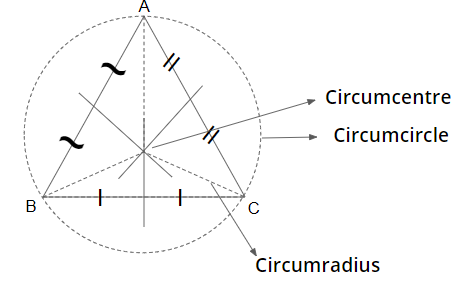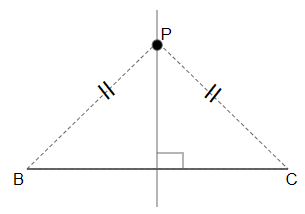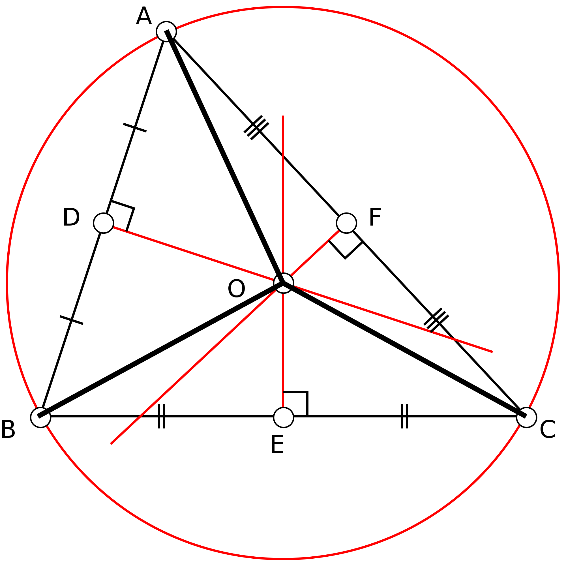# Circumcenter Formula

## Circumcenter Formulas- Definitions & Solved Examples

Circumcentre of a triangle is a unique point in the triangle where perpendicular bisectors of all three sides intersect. Circumcentre is also equidistant to all the vertices of the triangle.

Circumcentre lies inside for an acute-angled triangle, at the center of the hypotenuse for a right-angled triangle, and outside for an obtuse angled triangle.For better clarity of the circumcentre(Conceptual) of a triangle, let’s understand the concept of perpendicular bisector properly.If we construct a perpendicular bisector of seg. BC, then the arbitrary point P on the perpendicular bisector will be equidistant from the end points B, C of that segment.Now assume we are constructing perpendicular bisectors of all three sides of a triangle.Now as we can see pt. O is lying on all 3 perpendicular bisectors that means it will be equidistant from the end points of all 3 segments which are A, B, C.

So now we are clear about why we are constructing perpendicular bisectors for the circumcenter of a triangle.

* Calculate the circumcentre of a triangle, if coordinates of vertices are given.

1. Find the co-ordinates of mid-point of any one side of a triangle (let’s say, BC) using mid-point formula.
(x,y)={x1+x22,y1+y22}

Practice Question:
Find the coordinates of the circumcentre of a triangle whose vertices are (1,1) (3,4) and (5,-2).

Options
(a) (-5/6, 13/18)
(b) (-7/6, -11/18)
(c) (-5/6, -11/18)
(d) (5/6, -13/18)